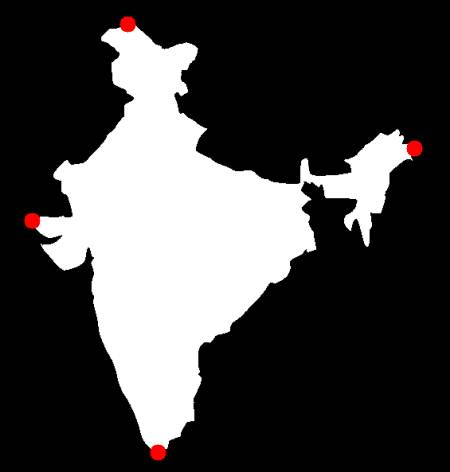# opencv-python 轮廓属性

## 目标：

• 学习提取一些常用的对象属性，如Solidity，Equivalent Diameter，Mask image，Mean Intensity

## 1. Aspect Ratio（长宽比）

$Aspect Ratio= frac{Width}{Height}$

x,y,w,h = cv.boundingRect(cnt)
aspect_ratio = float(w)/h


## 2. Extent（大小比）

$Extent= frac{Object Area}{Bounding Rectangle Area}$

area = cv.contourArea(cnt)
x,y,w,h = cv.boundingRect(cnt)
rect_area = w*h
extent = float(area)/rect_area


## 3. Solidity（密实比）

Solidity是轮廓区域与其凸包区域的比率。

$Solidity= frac{Contour Area}{Convex Hull Area}$

area = cv.contourArea(cnt)
hull = cv.convexHull(cnt)
hull_area = cv.contourArea(hull)
solidity = float(area)/hull_area


## 4. Equivalent Diameter（等效直径）

$Equivalent Diameter=sqrt{frac{4times Contour Area}{pi }}$

area = cv.contourArea(cnt)
equi_diameter = np.sqrt(4*area/np.pi)


## 5. Orientation（方向）

(x,y),(MA,ma),angle = cv.fitEllipse(cnt)


## 6. Mask & Pixel Points（掩模和像素点）

mask = np.zeros(imgray.shape,np.uint8)


## 7. 最大值，最小值及其位置

min_val, max_val, min_loc, max_loc = cv.minMaxLoc(imgray,mask = mask)


## 8. 平均颜色或平均灰度

mean_val = cv.mean(im,mask = mask)


## 9. 极点

leftmost = tuple(cnt[cnt[:,:,0].argmin()])
rightmost = tuple(cnt[cnt[:,:,0].argmax()])
topmost = tuple(cnt[cnt[:,:,1].argmin()])
bottommost = tuple(cnt[cnt[:,:,1].argmax()])